Most Affordable JEE | NEET | 8,9,10 Preparation by Kota's Top IITian Doctor Faculties

# NCERT Solutions for Class 11 Chemistry chapter 13 Hydrocarbons PDF - eSaral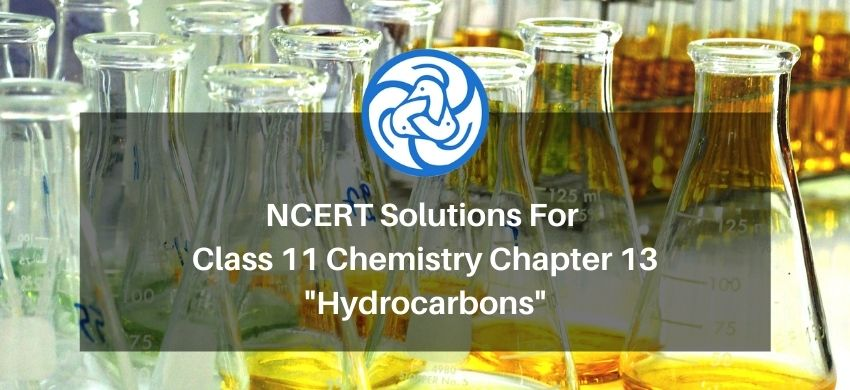# NCERT Solutions for Class 11 Chemistry chapter 13 Hydrocarbons PDF

Hey, are you a class 11 student and looking for ways to download NCERT Solutions for Class 11 Chemistry chapter 13 Hydrocarbons PDF? If yes. Then read this post till the end.

In this article, we have listed NCERT Solutions for Class 11 Chemistry chapter 13 Hydrocarbons PDF that are prepared by Kota’s top IITian’s Faculties by keeping Simplicity in mind.

If you want to learn and understand class 11 Chemistry chapter 13 "Hydrocarbons" in an easy way then you can use these solutions PDF.

NCERT Solutions helps students to Practice important concepts of subjects easily. Class 11 Chemistry solutions provide detailed explanations of all the NCERT questions that students can use to clear their doubts instantly.

If you want to score high in your class 11 Chemistry Exam then it is very important for you to have a good knowledge of all the important topics, so to learn and practice those topics you can use eSaral NCERT Solutions.

So, without wasting more time Let’s start.

### Download NCERT Solutions for Class 11 Chemistry chapter 13 Hydrocarbons PDF

Question 1. How do you account for the formation of ethane during chlorination of methane?

Solution: Chlorination of methane proceeds by free radical chain mechanism. The whole reaction takes place in three steps.

Step 1: chain Initiation

Step 2: chain Propagation

Step 3: chain termination

Step 1: Initiation: The reaction starts with the homolytic cleavage of Cl-Cl bond and free radical formed.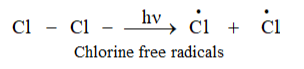Step 2: Propagation:

In the second step, chlorine-free radicals attack methane molecules and break down the C- H bond to generation methyl radicals occur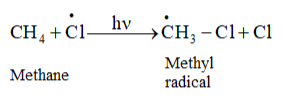These methyl radicals react with other chlorine-free radicals to form methyl chloride along with the liberation of a chlorine-free radical.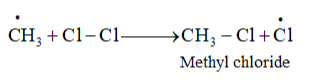Hence, methyl free radicals and chlorine-free radicals set up a chain reaction. While HCl and $\mathrm{CH}_{3} \mathrm{Cl}$ are the major products formed, other higher halogenated compounds are also formed as:

$\mathrm{CH}_{3} \mathrm{Cl}+\dot{\mathrm{C}} 1 \longrightarrow \dot{\mathrm{CH}}_{2} \mathrm{Cl}+\mathrm{HCl}$

$\dot{\mathrm{CH}}_{2} \mathrm{Cl}+\mathrm{Cl}-\mathrm{Cl} \longrightarrow \mathrm{CH}_{2} \mathrm{Cl}_{2}+\dot{\mathrm{C} 1}$

Step 3: Termination:

Formation of ethane is a result of the termination of chain reactions taking place as a result of the consumption of reactants as:

$\dot{\mathrm{C}} 1+\dot{\mathrm{C}} 1 \longrightarrow \mathrm{Cl}-\mathrm{Cl}$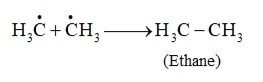Hence, by this process, ethane is obtained as a by-product of chlorination of methane.

Question 2. Write IUPAC names of the following compounds:

(a) $\mathrm{CH}_{3} \mathrm{CH}=\mathrm{C}\left(\mathrm{CH}_{3}\right)_{2}$

(b) $\mathrm{CH}_{2}=\mathrm{CH}-\mathrm{C} \equiv \mathrm{C}-\mathrm{CH}_{3}$

(c)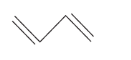(d)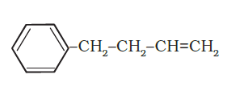(e)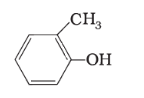(f)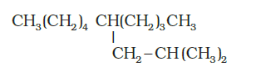(g)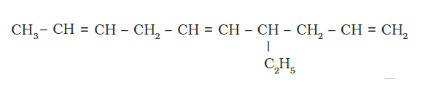Solution: (a)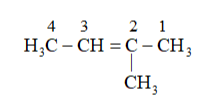Substituent : $\left(-C H_{3}\right)=$ methyl

Parent carbon chain(word root) $=4$ (bute)

Primary suffix $=$ ene

Secondary prefix $+$ primary prefix +word root $+$ Primary suffix $+$ seondary suffix

IUPAC name: 2-Methylbut-2-ene

(b)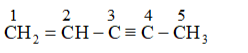Parent carbon chain(word root $)=5$ (pent)

Primary suffix $=$ (doube bond) ene, yne(triple bond)

Secondary prefix $+$ primary prefix +word root $+$ Primary suffix $+$ seondary suffix

IUPAC name: Pent 1 en 3 yne

(c)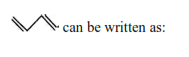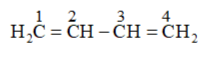Parent carbon chain(word root $)=4$ (bute)

Primary suffix $=$ (doube bond) ene

Secondary prefix $+$ primary prefix $+$ word root $+$ Primary suffix $+$ seondary suffix

IUPAC name: 1,3 -Butadiene or Buta-1,3-diene

(d)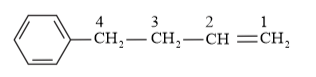IUPAC name: 4-Phenyl but-1-ene

(e)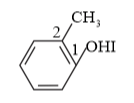IUPAC name: 2-Methyl phenol

(f)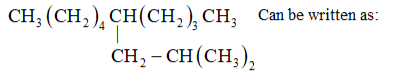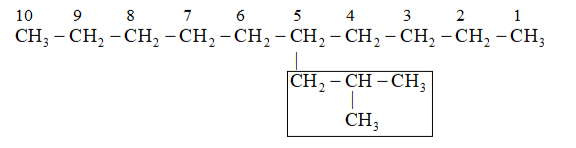Parent carbon chain(word root $)=10$ (deca)

Primary suffix $=$ ane

Substitute : (2-Methylpropyl)

Secondary prefix $+$ primary prefix +word root $+$ Primary suffix $+$ seondary suffix

IUPAC name: 5 -(2-Methylpropyl)-decane

(g)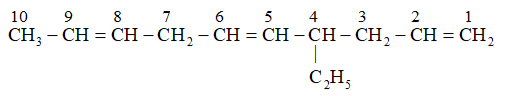Parent carbon chain(word root $)=10$ (deca)

Primary suffix =ene

Substitute : ethyl

Secondary prefix $+$ primary prefix $+$ word root $+$ Primary suffix $+$ seondary suffix

IUPAC name: 4-Ethyldeca-1, 5,8 -triene

Question 3. For the following compounds, write structural formulas and IUPAC names for all possible isomers having the number of double or triple bond as indicated:

(a) $\mathrm{C}_{4} \mathrm{H}_{8}$ (one double bond)

(b) $\mathrm{C}_{5} \mathrm{H}_{8}$ (one triple bond)

Solution: (a) The following structural isomers are possible for $\mathrm{C}_{4} \mathrm{H}_{8}$ with one double bond: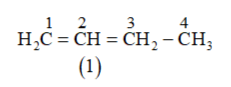IUPAC name of compound bute-1-ene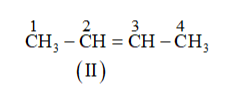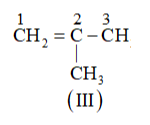IUPAC name of Compound is 2-Methylprop-1-ene.

(b) The following structural isomers are possible for $\mathrm{C}_{5} \mathrm{C}_{8}$ with one triple bond: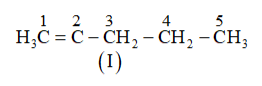IUPAC name of Compound is Pent-1-yne,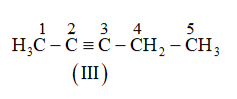IUPAC name of Compound is Pent-2-yne, and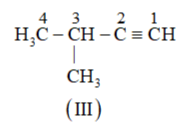The IUPAC name of Compound is 3 -Methylbut-1-ene.

Question 4. Write IUPAC names of the products obtained by the ozonolysis of the following compounds:

(i) Pent-2-ene

(ii) 3,4-Dimethylhept-3-ene

(iii) 2-Ethylbut-1-ene

(iv) 1-Phenylbut-1-ene

Solution: Oxidative ozonolysis:

Inpresence of $\mathrm{O}_{3} / \mathrm{H}_{2} \mathrm{O}, \mathrm{O}_{3} / \mathrm{Ag}_{2} \mathrm{O}, \mathrm{HCOOH}$ oxidative ozonolysis takes place.

Reductive ozonolysis:

In presence of $\mathrm{O}_{2} / \mathrm{H}_{2} \mathrm{O}, \mathrm{Zn}, \mathrm{O}_{3} /\left(\mathrm{CH}_{3}\right) \mathrm{S}, \mathrm{O}_{3} / \mathrm{PPb}_{3}, \mathrm{O}_{3} / \mathrm{LiAlH}_{4}$ or $\mathrm{NaBH}_{4}$ reductive ozonolysis takes place and products.

(i) Pent-2-ene undergoes ozonolysis as: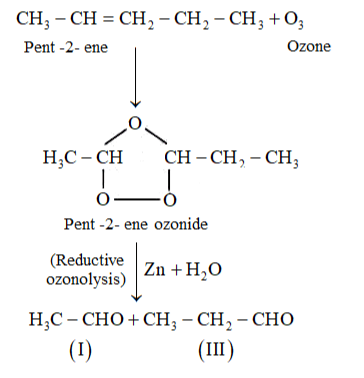The IUPAC name of Product (I) is ethanal and Product (II)is propanal.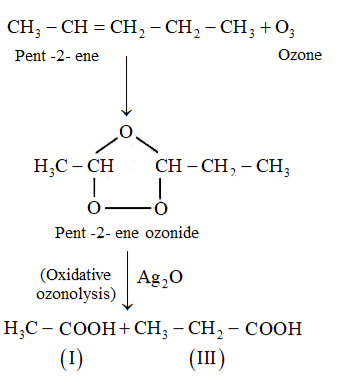The IUPAC name of Product (I) is ethanoic acid and Product (II)is propanoic acid

(ii) 3,4 -Dimethylhept- 3 -ene undergoes ozonolysis as: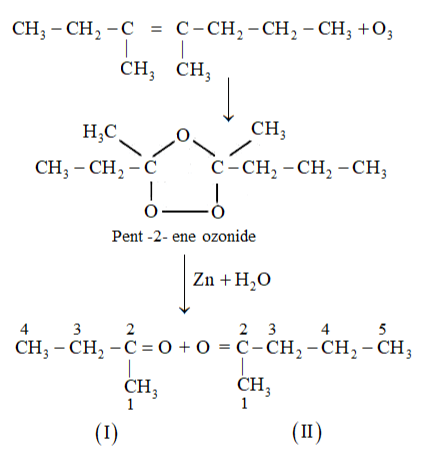The IUPAC name of Product (I)is butan-2-one and Product (II) is Pentan-2-one.

(iii) 2-Ethylbut-1-ene undergoes ozonolysis as: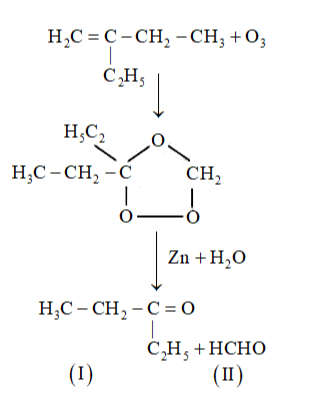The IUPAC name of Product (I)is pentan-3-one and Product (II)is methanal.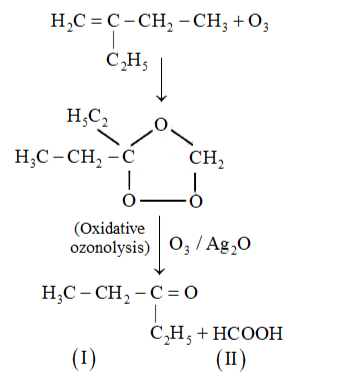The IUPAC name of Product (I)is pentan-3-one and Product (II)is methanoic acid.

(iv) 1-Phenylbut-1-ene undergoes ozonolysis as: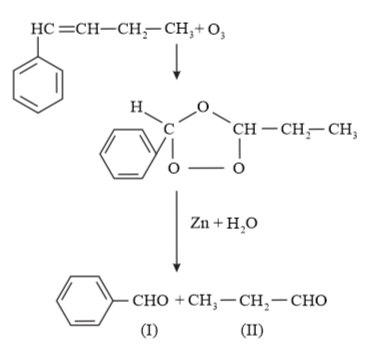The IUPAC name of Product (I)is benzaldehyde and Product (II)is propanal.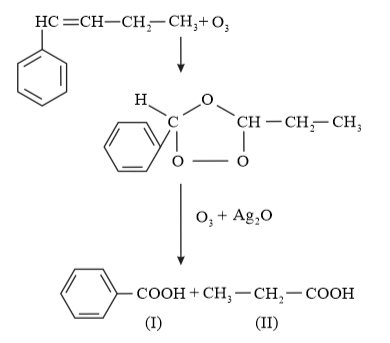The IUPAC name of Product (I)is benzoic acid and Product (II)is propanoic acid.

Question 5. An alkene ' $\mathrm{A}$ ' on ozonolysis gives a mixture of ethanal and pentan-3-one. Write structure and IUPAC name of 'A'.

Solution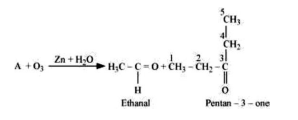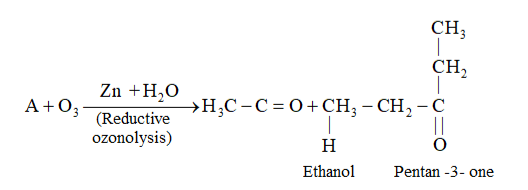During reductive ozonolysis, an ozonide having a cyclic structure is formed as an intermediate which undergoes cleavage to give the final products. Ethanal and pentan-3one are obtained from the intermediate ozonide. Hence, the expected structure of the ozonide is: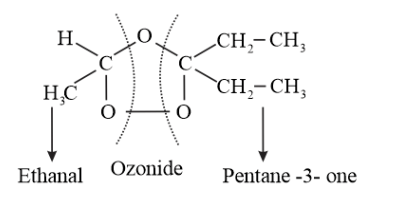This ozonide is formed as an addition of ozone to 'A'. The desired structure of 'A' can be obtained by the removal of ozone from the ozonide. Hence, the structural formula of 'A' is: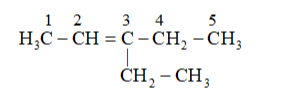The IUPAC name of ' $\mathrm{A}$ ' is 3 -Ethylpent-2-ene.

Question 6. An alkene 'A' contains three $\mathrm{C}-\mathrm{C}$, eight $\mathrm{C}-\mathrm{H} \sigma$ bonds and one $\mathrm{C}-\mathrm{C} \pi$ bond. 'A' on ozonolysis gives two moles of an aldehyde of molar mass $44 \mathrm{u}$. Write IUPAC name of $\cdot \mathrm{A}^{\prime}$

Solution: Given in question, 'A' on ozonolysis gives two moles of an aldehyde of molar mass $44 \mathrm{u}$. The formation of two moles of an aldehyde indicates the presence of identical structural units on both sides of the double bond containing carbon atoms. Hence, the structure of 'A' can be represented as:

$\mathrm{XC}=\mathrm{CX}$

There are eight $\mathrm{C}-\mathrm{H} \sigma$ bonds. Hence, there are 8 hydrogen atoms in ' $\mathrm{A}$ '. Also, there are three C-C bonds. Hence, there are four carbon atoms present in the structure of ' $A$ '. Combining the inferences, the structure of 'A' can be represented as: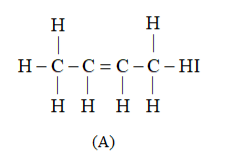'A' has $3 \mathrm{C}-\mathrm{C}$ bonds, $8 \mathrm{C}-\mathrm{H} \sigma$ bonds, and one $\mathrm{C}-\mathrm{C} \pi$ bond.

Hence, the IUPAC name of compound 'A' is But-2-ene.

Ozonolysis of 'A' takes place as: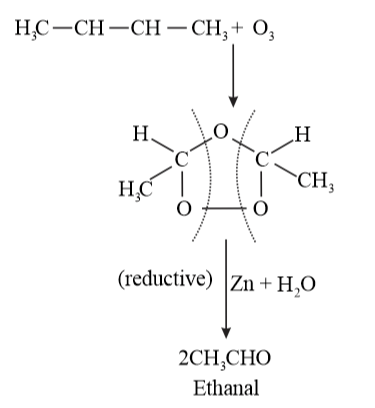The final product is ethanal with molecular mass

$=[(2 \times 12)+(4 \times 1)+(1 \times 16)]$

$=44 \mathrm{u}$

Question 7. Propanal and pentan-3-one are the ozonolysis products of an alkene? What is the structural formula of the alkene?

Solution: As per the given information, propanal and pentan-3-one are the ozonolysis products of an alkene. Let the given alkene be ' $\mathrm{A}$ '. Writing the reverse of the ozonolysis reaction, we get: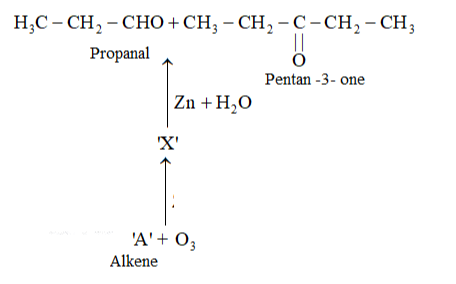The products are obtained on the cleavage of ozonide ' $\mathrm{X}$ '. Hence, ' $\mathrm{X}$ ' contains both products in the cyclic form. The possible structure of ozonide can be represented as: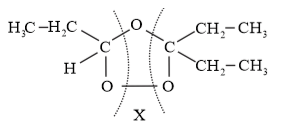Now, 'X' is an addition product of alkene 'A' with ozone. Therefore, the possible structure of alkene ' $\mathrm{A}$ ' is: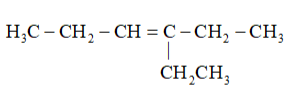Question 8. Write chemical equations for combustion reaction of the following hydrocarbons:

(i) Butane

(ii) Pentene

(iii) Hexyne

(iv) Toluene

Solution: Combustion of hydrocarbon with $O_{2}$ gives product $\mathrm{CO}_{2}(\mathrm{~g})$ and $\mathrm{H}_{2} \mathrm{O}(\mathrm{l})$ at temperature below $100^{\circ} \mathrm{C}$ and form $\mathrm{CO}_{2}(\mathrm{~g})$ and $\mathrm{H}_{2} \mathrm{O}(\mathrm{g})$ at temperature of $100^{\circ} \mathrm{C}$.

(i)

$2 \mathrm{C}_{4} \mathrm{H}_{10}(\mathrm{~g})+13 \mathrm{O}_{2}(\mathrm{~g}) \rightarrow 8 \mathrm{CO}_{2}(\mathrm{~g})$

$+10 \mathrm{H}_{2} \mathrm{O}(\mathrm{g})+$ Heat

Butane

(ii)

$2 \mathrm{C}_{5} \mathrm{H}_{10}(\mathrm{~g})+15 \mathrm{O}_{2}(\mathrm{~g}) \rightarrow 10 \mathrm{CO}_{2}(\mathrm{~g})$

$1+10 \mathrm{H}_{2} \mathrm{O}(\mathrm{g})+$ Heat

Pentene

(iii)

$2 \mathrm{C}_{6} \mathrm{H}_{10}(\mathrm{~g})+17 \mathrm{O}_{2}(\mathrm{~g}) \rightarrow 12 \mathrm{CO}_{2}(\mathrm{~g})$

$+10 \mathrm{H}_{2} \mathrm{O}(\mathrm{g})+$ Heat

Hexyne

(iv)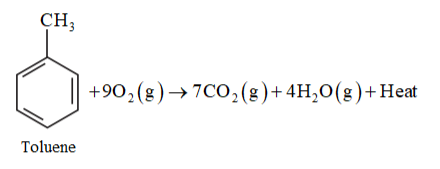Question 9. Draw the cis and trans structures of hex-2-ene. Which isomer will have higher b.p. and why?

Solution: Hex-2-ene is represented as:

$\mathrm{H}_{3} \mathrm{C}-\mathrm{HC}=\mathrm{CH}-\mathrm{CH}_{2}-\mathrm{CH}_{3}$

Geometrical isomers of hex-2-ene are: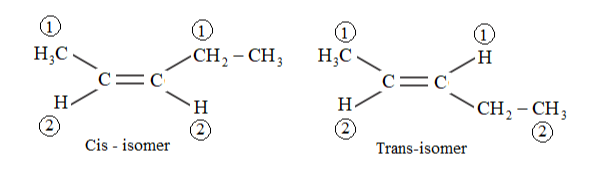Cis isomer: Higher priority group present at same side.

Trans: Higher priority group present at opposite side where 1 is higher priority group and 1 is lower priority group.

The dipole moment of cis-compound is a sum of the dipole moments of $\mathrm{C}-\mathrm{CH}_{3}$ and C- $\mathrm{CH}_{2} \mathrm{CH}_{3}$ bonds acting in the same direction.

The dipole moment of trans-compound is the resultant of the dipole moments of $\mathrm{C}-\mathrm{CH}_{3}$ and $\mathrm{C}-\mathrm{CH}_{2} \mathrm{CH}_{3}$ bonds acting in opposite directions.

Hence, cis-isomer is more polar than trans-isomer. The higher the polarity, the greater is the intermolecular dipole-dipole interaction and the higher will be the boiling point. Hence, cis-isomer will have a higher boiling point than trans-isomer.

Question 10. Why is benzene extra ordinarily stable though it contains three double bonds?

Solution: Benzene is a hybrid of resonating structures given as: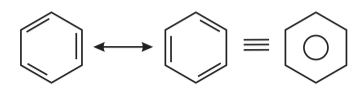All six carbon atoms in benzene are $s p^{2}$ hybridized. The two $s p^{2}$ hybrid orbitals of each carbon atom overlap with the $s p^{2}$ hybrid orbitals of adjacent carbon atoms to form six sigma bonds in the hexagonal plane. The remaining $s p^{2}$ hybrid orbital on each carbon atom overlaps with the $s$ -orbital of hydrogen to form six sigma C- H bonds. The remaining unhybridized $p$ -orbital of carbon atoms has the possibility of forming three $\pi$ bonds by the lateral overlap of

$\mathrm{C}_{1}-\mathrm{C}_{2}, \mathrm{C}_{3}-\mathrm{C}_{4}, \mathrm{C}_{5}-\mathrm{C}_{6}$, or $\mathrm{C}_{2}-\mathrm{C}_{3}, \mathrm{C}_{4}-\mathrm{C}_{5}, \mathrm{C}_{6}-\mathrm{C}_{1}$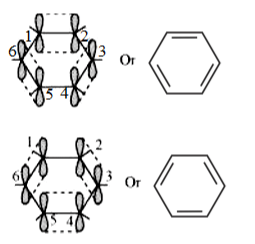The six $\pi$ 's are delocalized and can move freely about the six carbon nuclei. Even after the presence of three double bonds, these delocalized $\pi$ -electrons stabilize benzene.

Question 11. What are the necessary conditions for any system to be aromatic?

Solution: A compound is said to be aromatic if it satisfies the following three conditions:

(i) Compound should have a planar structure.

(ii) The $\pi$ -electrons of the compound are completely delocalized in the ring.

(iii) The total number of $\pi$ -electrons present in the ring should be equal to $(4 n+2)$,

where $n=0,1,2 \ldots$ etc. This is known as Huckel's rule.

Question 12. Explain why the following systems are not aromatic?

(1)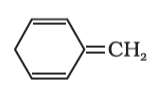(2)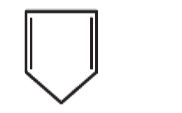(3)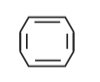Solution: (i)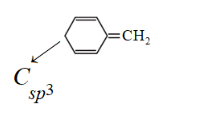Compund s not a planar becayse it has $C_{s p^{3}}$ which is tetrahedral. So, it non-aromatic compound.

(ii)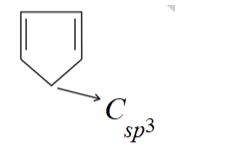Compund s not a planar becayse it has $C_{s p^{3}}$ which is tetrahedral. So, it non-aromatic compound.

(iii)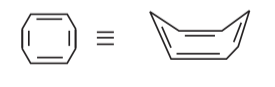The compound has tab type shape. So, it is non plannar hence it is non-aromatic compound.

Question 13. How will you convert benzene into

(i) $p$ -nitrobromobenzene

(ii) $m$ -nitrochlorobenzene

(iii) $p$ -nitrotoluene

(iv) acetophenone

Solution: (i) Benzene can be converted into $p$ -nitrobromobenzene as:

Benzene react with $B r_{2}$ inpresnece of lewis acid $F e B r_{3}$ and formed bromobenzene by electrophilic substitution reaction.

The $-B r$ shows $(-I$ and $+M)$ effect. Due to the $-1$ it is ring deactivating group towards electrophile attack and due to $+\mathrm{M}$ effect it is ortho/para directing group. So, $N O_{2}^{+}$ attack at ortho/para position of bromobezene.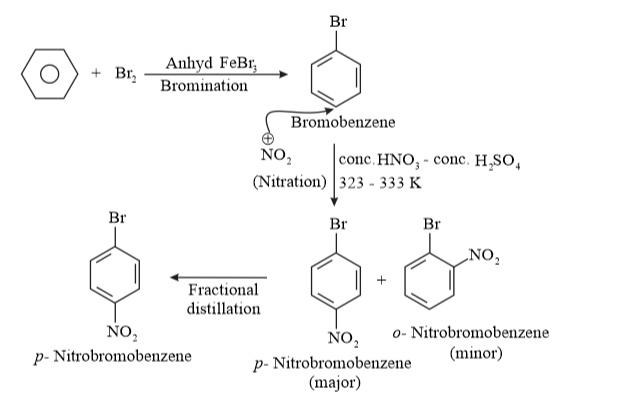(ii) Benzene can be converted into $m$ -nitrochlorobenzene as: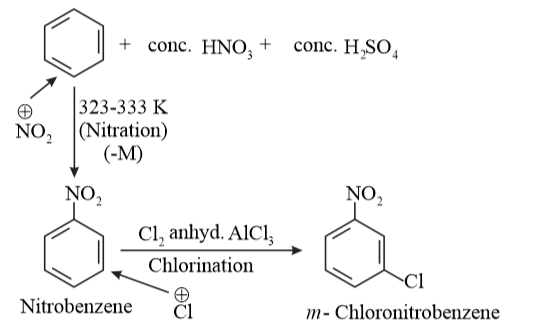$-N O_{2}$ group is $-M$ group. So, it is ring deactivating and meta directing group. So, electrophilic $C l^{+}$ attack at meta position.

(iii) Benzene can be converted into $p$ -nitrotoulene as: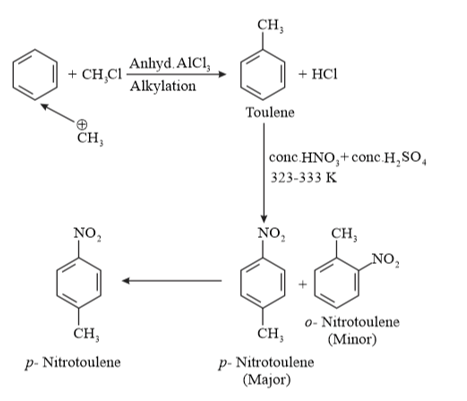$-C H_{3}$ is $+H>+I$, it is ring activating and ortho/para directing group. So, electrophile

(iv) Benzene can be converted into acetophenone as: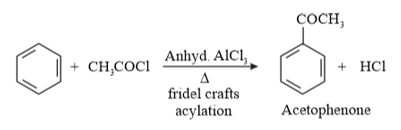$\mathrm{CH}_{3} \mathrm{CO}^{+}$ is electrophile is fridel crafts acetylation reaction.

Question 14. In the alkane $\mathrm{H}_{3} \mathrm{C}-\mathrm{CH}_{2}-\mathrm{C}\left(\mathrm{CH}_{3}\right)_{2}-\mathrm{CH}_{2}-\mathrm{CH}\left(\mathrm{CH}_{3}\right)_{2}$, identify $1^{\circ}, 2^{\circ}, 3^{\circ}$ carbon atoms and give the number of $\mathrm{H}$ atoms bonded to each one of these.

Solution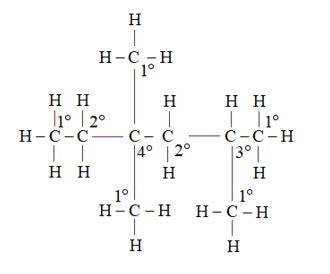$1^{\circ}$ carbon atoms are those which are bonded to only one carbon atom i.e., they have only one carbon atom as their neighbour. The given structure has five $1^{\circ}$ carbon atoms and fifteen hydrogen atoms attached to it.

$2^{\circ}$ carbon atoms are those which are bonded to two carbon atoms i.e., they have two carbon atoms as their neighbours. The given structure has two $2^{\circ}$ carbon atoms and four hydrogen atoms attached to it.

$3^{\circ}$ carbon atoms are those which are bonded to three carbon atoms i.e., they have three carbon atoms as their neighbours. The given structure has one $3^{\circ}$ carbon atom and only one hydrogen atom is attached to it.

Question 15. What effect does branching of an alkane chain has on its boiling point?

Solution. Alkanes has inter-molecular Van der Waals forces. The stronger the force, the greater will be the boiling point of the alkane.

Van der waals forces $\propto$ molecular weight

Compounds has same moleucular mass one has straight and another has branced structure.

As branching increases, the surface area of the molecule decreases which results in a small area of contact. As a result, the Van der Waals force also decreases which can be

overcome at a relatively lower temperature. Hence, the boiling point of an alkane chain decreases with an increase in branching.

Boiling point $\propto \frac{1}{\text { brancing }}$

Question 16. Addition of $\mathrm{HBr}$ to propene yields 2 -bromopropane, while in the presence of benzoyl peroxide, the same reaction yields 1-bromopropane. Explain and give mechanism.

Solution: Addition of HBr to propene is an example of an electrophilic substitution reaction. Hydrogen bromide provides an electrophile, $\mathrm{H}^{+} .$ This electrophile attacks the double bond to form $1^{\circ}$ and $2^{\circ}$ carbocations as shown:

Propene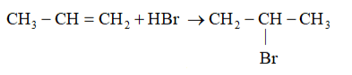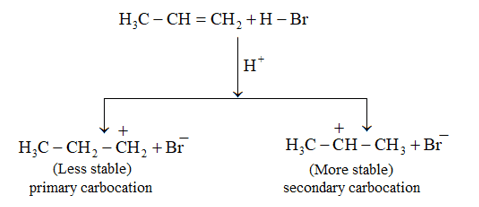Secondary carbocations are more stable than primary carbocations. Hence, the former predominates since it will form at a faster rate. Thus, in the next step, $\mathrm{Br}^{-}$ attacks the carbocation to form 2 -bromopropane as the major product.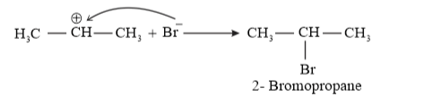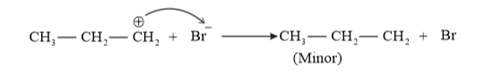This reaction follows Markovnikov's rule where the negative part of thereagent addendum is attached to the carbon atom having a lesser number of hydrogen atoms. While in the presence of benzoyl peroxide, an addition reaction takes place anti Markovnikov's rule. The reaction follows a free radical mechanism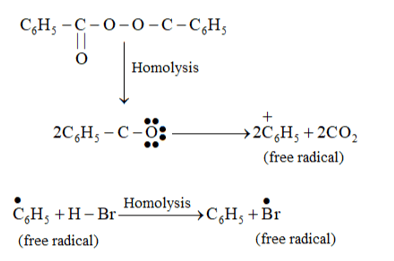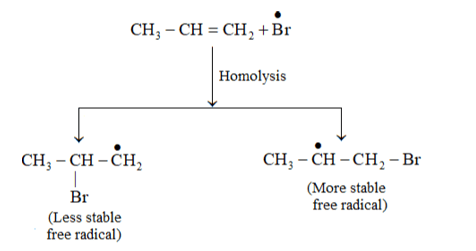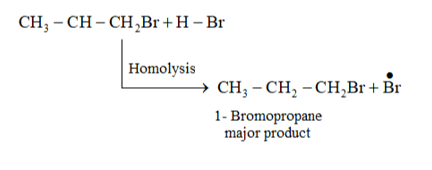In the presence of peroxide, Br free radical acts as an electrophile. Hence, two different products 2 bromopropane and 1-bromopropane are obtained on addition of $\mathrm{HBr}$ to propene in the absence and presence of peroxide respectively.

Question 17. Write down the products of ozonolysis of 1,2 -dimethylbenzene (o - xylene). How does the result support Kekulé structure for benzene?

Solution: $o$ - xylene has two resonance structures: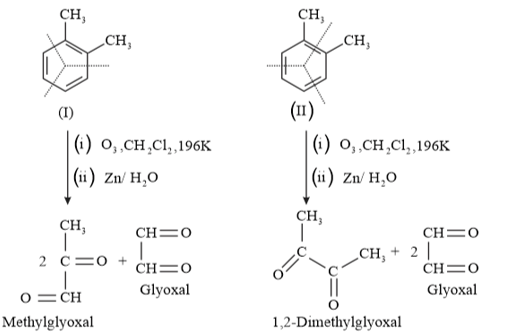All three products, i.e., methyl glyoxal, 1, 2-demethylglyoxal, and glyoxal are obtained from two Kekule structures. Since all three products cannot be obtained from any one of the two structures, this proves that $o$ -xylene is a resonance hybrid of two Kekule structures.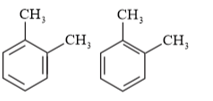Question 18. Arrange benzene, $n$ -hexane and ethyne in decreasing order of acidic behaviour. Also give reason for this behaviour.

Solution: Acidic strength $\propto$ stability of conjugate base (Anion)

Stability of conjugate base $\propto \frac{1}{\text { electron density }}$

Stability of conjugate base $\propto \frac{1}{\text { electron density }}$

Acidic character of a species is defined on the basis of ease with which it can lose its $\mathrm{H}^{-}$ atoms.

The hybridization state of carbon in the given compound is: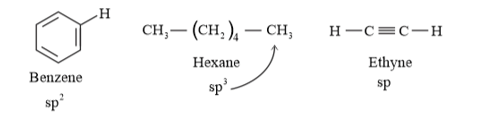Conjugate base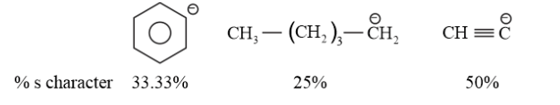As the s-character increases, the electronegativity of carbon increases and the electrons of $\mathrm{C}-\mathrm{H}$ bond pair lie closer to the carbon atom. As a result, partial positive charge of $\mathrm{H}^{-}$ atom increases and $\mathrm{H}^{+}$ ions are set free.

The s-character increases in the order: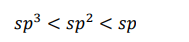Hence, the decreasing order of acidic behaviour is Ethyne > Benzene > Hexane.

Question 19. Why does benzene undergo electrophilic substitution reactions easily and nucleophilic substitutions with difficulty?

Solution: Benzene is a planar molecule having delocalized electrons above and below the plane of ring. Hence, it is electron-rich. As a result, it is highly attractive to electron deficient species i.e., electrophiles and $\mathrm{H}^{+}$ is good leaving group.

Therefore, it undergoes electrophilic substitution reactions very easily.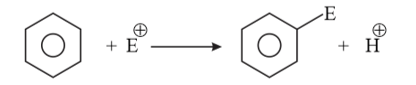Nucleophiles are electron-rich. Hence, they are repelled by benzene. $H^{-}$ (hydride) is poor leaving group. Hence, benzene undergoes nucleophilic substitutions with difficulty.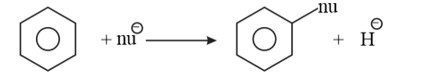Question 20. How would you convert the following compounds into benzene?

(i) Ethyne

(ii) Ethene

(iii) Hexane

Solution: (i) Benzene from Ethyne: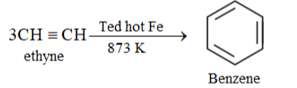(ii) Benzene from Ethene: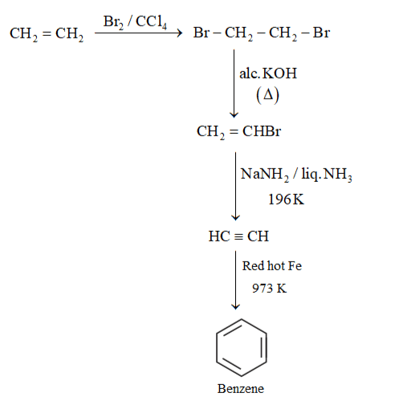(iii) Hexane to Benzene: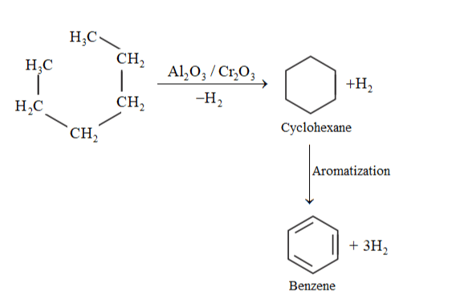When alkene (carbon contain 6 or more than 6 ) heated at hight temperature and high pressure inpresence of $\mathrm{Al}_{2} \mathrm{O}_{3}, \mathrm{Cr}_{2} \mathrm{O}_{3}$ undergoes aromatization.

Question 21. Write structures of all the alkenes which on hydrogenation give 2 -methylbutane.

Solution: Structure of 2-methylbutane is:

By the hydrogenation of alkene the skeleton wull not change so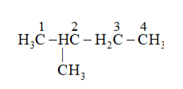On the basis, various alkenes that will give 2-methylbutane on hydrogenation are:

(a) 3 -methyl butane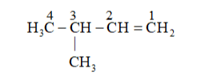(b) 2 -methyl but-2-ene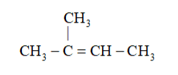(c) 2 -methyl butene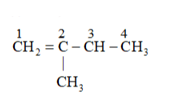Question 22. Arrange the following set of compounds in order of their decreasing relative reactivity with an electrophile, $\mathrm{E}^{+}$

(a) Chlorobenzene, 2,4 -dinitrochlorobenzene, $p$ -nitrochlorobenzene

(b) Toluene, $p-\mathrm{H}_{3} \mathrm{C}-\mathrm{C}_{6} \mathrm{H}_{4}-\mathrm{NO}_{2}, p-\mathrm{O}_{2} \mathrm{~N}-\mathrm{C}_{6} \mathrm{H}_{4}-\mathrm{NO}_{2}$

Solution: Electrophiles are reagents that participate in a reaction by accepting an electron pair in order to bond to nucleophiles.

The higher the electron density on a benzene ring, the more reactive is the compound towards an electrophile, $\mathrm{E}^{+}$ (Electrophilic reaction).

(a) The presence of an electron withdrawing group deactivates the aromatic ring by decreasing the electron density at ortho and para.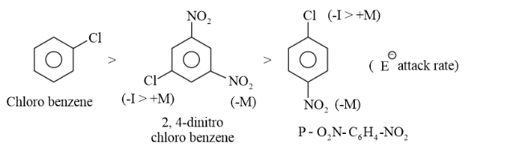$-N O_{2}$ group is more electron with drawing (due to $+M$ or mesomeric effect) group than $-C l$ group. Because $-C l$ show $-I$ effect and $+M$ effect also. $-I$ is feeable than $-M$ effect so, $C l$ decrease less electron density as compare to $-N O_{2}$ group.

Chlorobenzene $>\mathrm{p}$ - nitrochlorobenzene $>2,4$ -dinitrochlorobenzene

(b) While $-\mathrm{CH}_{3}$ is an electron donating group, $\mathrm{NO}_{2}^{-}$ group is electron withdrawing. Hence, toluene will have the maximum electron density and is most easily attacked by $\mathrm{E}^{+} . \mathrm{NO}_{2}^{-}$ is an electron withdrawing group. Hence, when the number of $\mathrm{NO}_{2}^{-}$ substituents is greater, the order is as follows:

Toluene $>p-\mathrm{CH}_{3}-\mathrm{C}_{6} \mathrm{H}_{4}-\mathrm{NO}_{2}, p-\mathrm{O}_{2} \mathrm{~N}-\mathrm{C}_{6} \mathrm{H}_{4}-\mathrm{NO}_{2}$

Question 23. Out of benzene, $m$ -dinitrobenzene and toluene which will undergo nitration most easily and why?

Solution: Rate of nitration depends on the presence of electron density on the compound to form nitrates. Nitration reactions are examples of electrophilic substitution reactions where an electron-rich species is attacked by a nitronium ion $\left(\mathrm{NO}_{2}^{+}\right) / E^{+}$

$-C H_{3}$ is ring activating group and $-N O_{2}$ is ring deactivating group.

Now, $\mathrm{CH}_{3}$ group is electron donating and $\mathrm{NO}_{2}^{-}$ is electron withdrawing. Therefore, toluene will have the maximum electron density among the three compounds followed by benzene.

On the other hand, $\mathrm{m}$ - Dinitrobenzene will have the least electron density. Hence, it will undergo nitration with difficulty. Hence, the increasing order of nitration is as follows: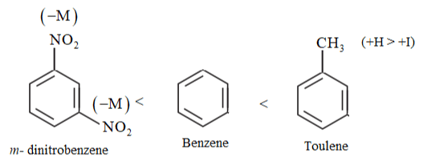Rate of nitration by electrophilic substitution.

Question 24. Suggest the name of a Lewis acid other than anhydrous aluminium chloride which can be used during ethylation of benzene.

Solution: The ethylation reaction of benzene involves the substitution of an ethyl group (electrophile) on the benzene ring. Such a reaction is called a Friedel-Craft alkylation reaction. This reaction takes place in the presence of a Lewis acid.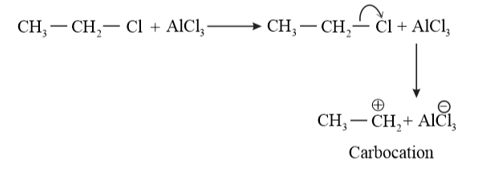Any Lewis acid like anhydrous $\mathrm{FeCl}_{3}, \mathrm{SnCl}_{2}, \mathrm{BF}_{3}$ etc. can be used during the ethylation of benzene.

Question 25. Why is Wurtz reaction not preferred for the preparation of alkanes containing odd number of carbon atoms? Illustrate your answer by taking one example.

Solution: Wurtz reaction is limited for the synthesis of symmetrical alkanes (alkanes with an even number of carbon atoms) In the reaction, two similar alkyl halides are taken as reactants and an alkane, containing double the number of carbon atoms, are formed.

Example: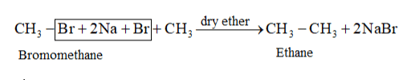Wurtz reaction cannot be used for the preparation of unsymmetrical alkanes because if two dissimilar alkyl halides are taken as the reactants, then a mixture of alkanes is obtained as the products. Since the reaction involves free radical species, a side reaction also occurs to produce an alkene. For example, the reaction of bromomethane and iodoethane gives a mixture of alkanes.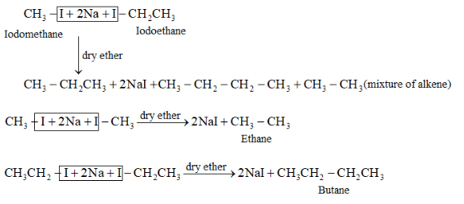The boiling points of alkanes (obtained in the mixture) are very close. Hence, it becomes difficult to separate them.